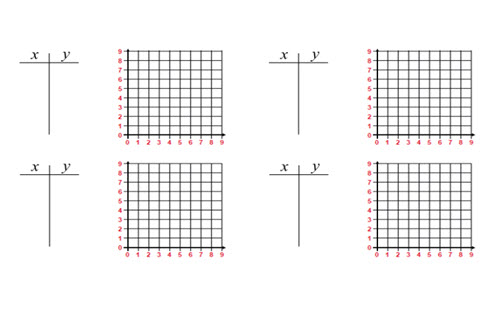Go to website

# Straight line?

The sequence of lessons explores students' capacity to utilise a rule, to rearrange equations and determine angle of elevation. The third lesson introduces a third variable, introducing students to three-dimnesional coordinates, teaching students how to plot points and read points on a Cartesian grid. Guidance is provided on how to interpret student responses to diagnose misconceptions and includes follow-up lessons to address these. Teacher notes and resources are also provided for use throughout the lessons.

Year level(s) Year 8
Audience Teacher, Student
Purpose Assessment task, Curriculum design, Content knowledge, Student task, Teaching resource, Teaching strategies
Teaching strategies and pedagogical approaches Collaborative learning, Differentiated teaching, Explicit teaching, Mathematics investigation, Setting goals, Structuring lessons, Worked examples
Keywords linear equations, Cartesian planes, y axis, x axis, substitute, gradient, y intercept, x intercept, positive, negative

## Curriculum alignment

Curriculum connections ICT general capability, Numeracy
Strand and focus Algebra, Number, Apply understanding, Build understanding
Topics Addition and subtraction, Algebraic expressions, Data representation and interpretation, Integers, Linear relationships, Multiplication and division
AC: Mathematics (V9.0) content descriptions
AC9M8A02
Graph linear relations on the Cartesian plane using digital tools where appropriate; solve linear equations and one-variable inequalities using graphical and algebraic techniques; verify solutions by substitution

Numeracy progression Number patterns and algebraic thinking (P7)